# Newton diagram

Newton polygon

A convex polygonal line, introduced by I. Newton in 1669 (see ) to determine the exponents of the principal terms of algebraic functions. The process of finding successively the terms of the expansion of an algebraic function with the help of the Newton diagram is called the method of the Newton diagram. In more detail it was worked out by V. Puiseux  and it is sometimes called the Puiseux diagram in mathematical literature. Before Puiseux, an algebraic version of the Newton diagram was studied by J.L. Lagrange .

Letbe a pseudo-polynomial in, that is, letwhere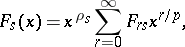andare complex variables,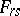are complex numbers,is a natural number,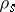are non-negative rational numbers,, and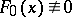. As a rule one assumes that if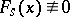, then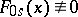, hence,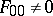,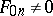. A solutionof the equation(1)

is sought for in the form of a series(2)

whereor, briefly,,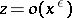as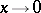. To determine the possible values ofandone substitutes (2) in (1), collects terms with equal powers of, and equates to zero the coefficients of these powers.

The process begins with the term of lowest degree. As long as the exponentis not yet determined, there is no way of telling which of the resulting terms are lowest in. However, the terms of lowest order are among the following:(3)

whereranges over those values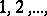for which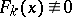. To annihilate the terms of lowest order one has to chooseso that at least two of the exponents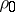,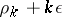,coincide and the remaining ones are to be not smaller. This argument leads to Newton's diagram.

In the plane one takes a rectangular Cartesian coordinate system and plots the points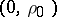,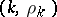and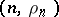, whereranges over the same values as in (3). Through the pointone draws the line that coincides with the-axis and then rotates aroundanti-clockwise until it falls onto one of the plotted points, say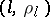. The tangent of the angle that the linepassing throughandmakes with the negative-axis is one of the values, since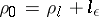, and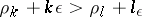if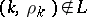. Suppose thatis the point onwith largest-coordinate and thatis rotated anti-clockwise around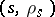until it falls onto another one of the plotted points, say,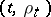with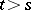. Let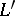be the line throughand. The tangent of the angle betweenand the negative-axis gives another possible value of. Continuing these constructions one obtains a convex polygonal line, which is called Newton's diagram.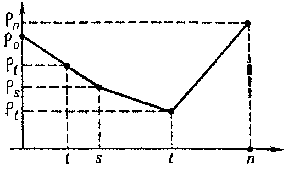Figure: n066520a

The value of the coefficient ofis determined as follows. Let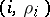and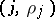be the extreme points of a segment of the Newton diagram that determines one of the possible values of. To annihilate the terms of lowest order when (2) is substituted in (1) it is necessary and sufficient that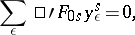(4)

where the prime in the sum denotes that summation is over thosefor which. Equation (4) has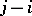non-zero roots (including multiplicity), that is, as many as the length of the projection of the relevant segment of the Newton diagram. Hence it is clear that by the method of the Newton diagram one obtains allvalues of the principal termin (2). By the same method one determines the next term in the expansion (2), etc. As a result, allsolutions of (1) have the form (2), so-called Puiseux series (see Algebraic function). The method of the Newton diagram is also applicable to the solution of differential equations.